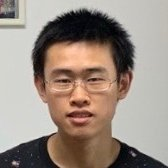Timezone: »

Poster
Independence Testing for Bounded Degree Bayesian Networks
Arnab Bhattacharyya · Clément L Canonne · Qiping Yang

Tue Nov 29 02:00 PM -- 04:00 PM (PST) @ Hall J #818
We study the following independence testing problem: given access to samples from a distribution $P$ over $\{0,1\}^n$, decide whether $P$ is a product distribution or whether it is $\varepsilon$-far in total variation distance from any product distribution. For arbitrary distributions, this problem requires $\exp(n)$ samples. We show in this work that if $P$ has a sparse structure, then in fact only linearly many samples are required.Specifically, if $P$ is Markov with respect to a Bayesian network whose underlying DAG has in-degree bounded by $d$, then $\tilde{\Theta}(2^{d/2}\cdot n/\varepsilon^2)$ samples are necessary and sufficient for independence testing.

#### Author Information

##### Qiping Yang (National University of Singapore)Research Assistant at National University of Singapore. Research interests: statistics, learning theory and algorithms.